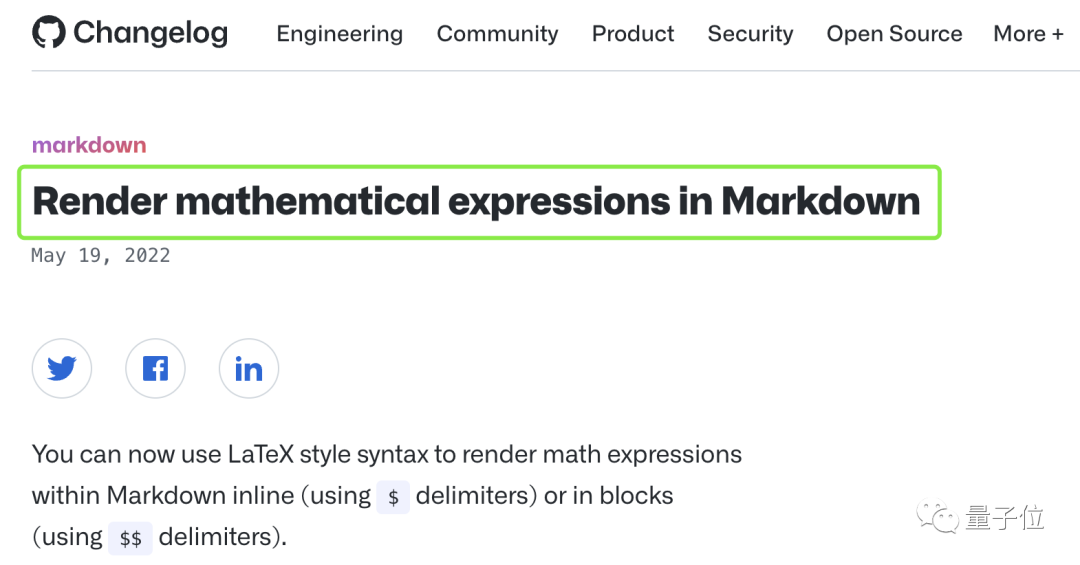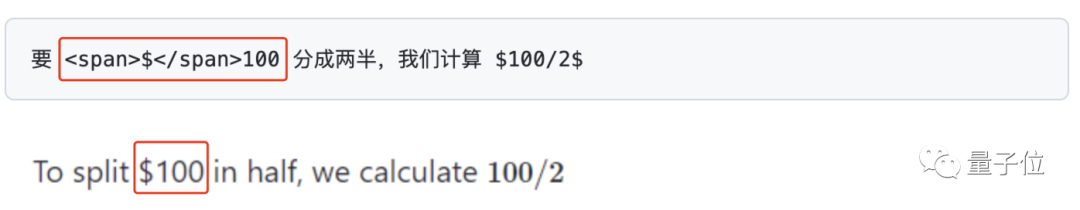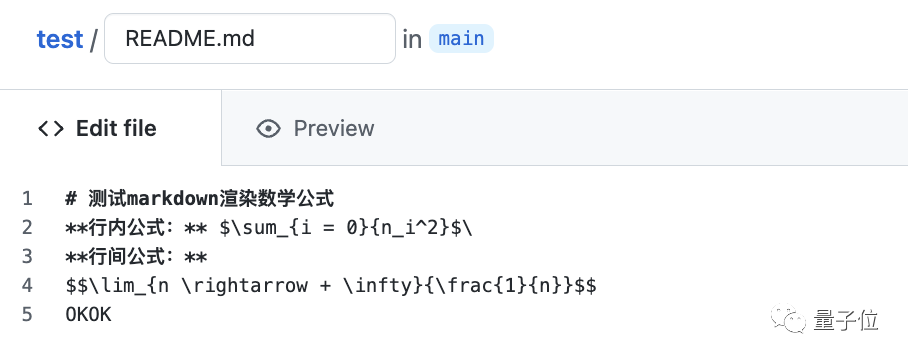# GitHub支持用LaTeX写数学公式了！亲测有效

……This sentence uses $delimiters to show math inline:$\sqrt{3x-1}+(1+x)^2$然后就能直接正确显示公式了：# 2、编写行间公式 如果你想在行间插入公式，只需另起一行并用两个“$”进行分隔：

The Cauchy-Schwarz Inequality

$$\left( \sum{k=1}^n a_k b_k \right)^2 \leq \left( \sum{k=1}^n ak^2 \right) \left( \sum{k=1}^n b_k^2 \right)$$（1）如果你的公式包含“$”，则需要在前面加上“\”进行转义；（2）如果在公式之外你的正文也包含“$”，则需要用“<span></span>”标记；